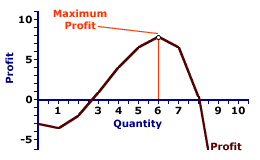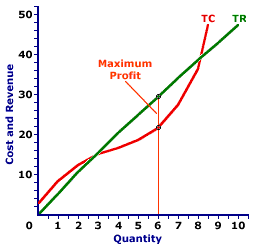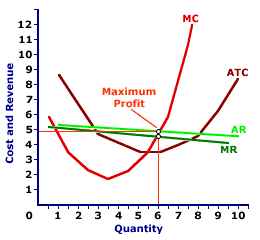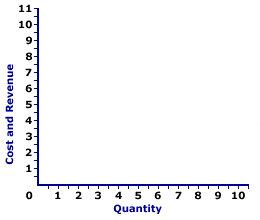Monday  February 24, 2020
 AmosWEB means Economics with a Touch of Whimsy!MARGINAL FACTOR COST CURVE, PERFECT COMPETITION: A curve that graphically represents the relation between marginal factor cost incurred by a perfectly competitive firm for hiring an input and the quantity of input employed. A profit-maximizing perfectly competitive firm hires the quantity of input found at the intersection of the marginal factor cost curve and marginal revenue product curve. The marginal factor cost curve for a perfectly competitive firm with no market control is horizontal.MONOPOLISTIC COMPETITION, PROFIT MAXIMIZATION:

A monopolistically competitive is presumed to produce the quantity of output that maximizes economic profit--the difference between total revenue and total cost. This production decision can be analyzed directly with economic profit, by identifying the greatest difference between total revenue and total cost, or by the equality between marginal revenue and marginal cost.
Profit Maximization
Profit CurveTotal CurvesMarginal CurvesThe profit-maximizing level of output is a production level that achieves the greatest level of economic profit given existing market conditions and production cost. For a monopolistically competitive firm, this entails adjusting the price and corresponding production level to achieved the desired match between total revenue and total cost.

### Three Views

Profit-maximizing output can be identified in one of three ways--directly with economic profit, with a comparison of total revenue and total cost, and with comparison of marginal revenue and marginal cost.

This exhibit illustrates how it can be identified for a monopolistically competitive firm, such as that operated by Manny Mustard's House of Sandwich, a well-known monopolistically competitive supplier of Deluxe Club Sandwiches, a tasty luncheon treat enjoyed by many hungry Shady Valley residents. Manny is one of many firms that produce sandwiches and similar products, meaning he faces a relatively elastic negatively-sloped demand curve.

The top panel presents the profit curve. The middle panel presents total revenue and total cost curves. The bottom panel presents marginal revenue and marginal cost curves. In all three panels, Manny maximizes profit when producing 6 sandwiches.

• Profit: First, profit maximization can be illustrated with a direct evaluation of profit. If the profit curve is at its peak, then profit is maximized. In the top panel, the profit curve achieves its highest level at 6 sandwiches. At other output levels, profit is less.

• Total Revenue and Total Cost: Second, profit maximization can be identified by a comparison of total revenue and total cost. The quantity of output that achieves the greatest difference of total revenue over total cost is profit maximization. In the middle panel, the vertical gap between the total revenue and total cost curves is the greatest at 6 sandwiches. For smaller or larger output levels, the gap is either less or the total cost curve lies above the total revenue curve.

• Marginal Revenue and Marginal Cost: Third, profit maximization can be identified by a comparison of marginal revenue and marginal cost. If marginal revenue is equal to marginal cost, then profit cannot be increased by changing the level of production. Increasing production adds more to cost than revenue, meaning profit declines. Decreasing production subtracts more from revenue than from cost, meaning profit also declines. In the bottom panel, the marginal revenue and marginal cost curves intersect at 6 sandwiches. At larger or smaller output levels, marginal cost exceeds marginal revenue or marginal revenue exceeds marginal cost.

### More on the Marginal View

Further analysis of the marginal approach to analyzing profit maximization provides further insight into the short-run production decision of a monopoly.

First, consider the logic behind using marginals to identify profit maximization.

1. Marginal revenue indicates how much total revenue changes by producing one more or one less unit of output.

2. Marginal cost indicates how much total cost changes by producing one more or one less unit of output.

3. Profit increases if marginal revenue is greater than marginal cost and profit decreases if marginal revenue is less than marginal cost.

4. Profit neither increases nor decreases if marginal revenue is equal to marginal cost.

5. As such, the production level that equates marginal revenue and marginal cost is profit maximization.
Profit Maximization,
The Marginal ViewWith this in mind, now consider this exhibit to the right, which will eventually contain the marginal revenue and marginal cost curves for Manny Mustard's sandwich production.
• Average Revenue: First up is the average revenue curve, which can be seen with a click of the [Average Revenue] button. Because Manny Mustard is a monopolistically competitive firm, this average revenue curve is also its negatively-sloped demand curve.

• Marginal Revenue: A click of the [Marginal Revenue] button reveals the green line labeled MR that depicts the marginal revenue Manny receives from sandwich production. Because Manny is a price maker, this marginal revenue curve is also a negatively-sloped line, and it lies beneath the average revenue (and demand) curve. However, because Manny's demand relatively elastic, these two curves are close together.

• Marginal Cost: A click of the [Marginal Cost] button reveals a red U-shaped curve labeled MC that represents the marginal cost Manny incurs in the production of sandwiches. The shape is based on increasing, then decreasing marginal returns.

• Profit Maximization: Profit is maximized at the quantity of output found at the intersection of the marginal revenue and marginal cost curves, which is 6 sandwiches. Click the [Profit Max] button to highlight this production level. This is the same profit-maximizing level identified using the total revenue and total cost curves and the profit curve.
Consider what results if marginal revenue is not equal to marginal cost:

• If marginal revenue is greater than marginal cost, as is the case for small quantities of output, then the firm can increase profit by increasing production. Extra production adds more to revenue than to cost, so profit increases.

• If marginal revenue is less than marginal cost, as is the case for large quantities of output, then the firm can increase profit by decreasing production. Reducing production reduces revenue less than it reduces cost, so profit increases.

• If marginal revenue is equal to marginal cost, then the firm cannot increase profit by producing more or less output. Profit is maximized.
Once the profit maximizing output is revealed, the last step is to identify the price charged by Manny. This is easily accomplished by clicking the [Price] button. Price is found by extending the 6-sandwich quantity upward to the average revenue curve, which is the demand curve. Buyers are willing to pay \$4.95 per sandwich if 6 sandwiches are sold.

Note that this \$4.95 price is greater than the \$4.65 marginal cost, which indicates that monopolistic competition does not technically achieve the price-equals-marginal-cost condition for efficiency. However, because the demand curve is relatively elastic, the difference is small.

 <= MONOPOLISTIC COMPETITION, PROFIT ANALYSIS MONOPOLISTIC COMPETITION, REVENUE DIVISION =>Recommended Citation:

MONOPOLISTIC COMPETITION, PROFIT MAXIMIZATION, AmosWEB Encyclonomic WEB*pedia, http://www.AmosWEB.com, AmosWEB LLC, 2000-2020. [Accessed: February 24, 2020].

Check Out These Related Terms...

Or For A Little Background...

And For Further Study...
Search Again?GRAY SKITTERY[What's This?] Today, you are likely to spend a great deal of time at a crowded estate auction looking to buy either a video game player or an AC adapter that won't fry your computer. Be on the lookout for high interest rates.Your Complete ScopeThe earliest known use of paper currency was about 1270 in China during the rule of Kubla Khan."In war, there is no second prize for the runner-up."-- Omar Bradley, US Army generalTGETokyo Grain Exchange (Japan)A PEDestrian's Guide Xtra CreditTell us what you think about AmosWEB. Like what you see? Have suggestions for improvements? Let us know. Click the User Feedback link.| | | | | | | | | | |
| | | |

Thanks for visiting AmosWEB